Question

# The figure below shows an initially stationary block of mass m on a floor

The figure below shows an initially stationary block of mass m on a floor. A force of magnitude F = 0.550mg is then applied at upward angle θ = 24°.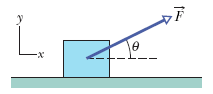(a) What is the magnitude of the acceleration of the block across the floor if the friction coefficients are μs = 0.610 and μk = 0.505?

(b) What is the magnitude of the acceleration of the block across the floor if the friction coefficients are μs = 0.395 and μk = 0.295?

What is the vertical component and horizontal component of the applied force? What is the gravitational force? What is the vertical acceleration? From Newton's second law for vertical motion, what is the normal force? What is fs,max? How does that value compare with the horizontal component of the applied force?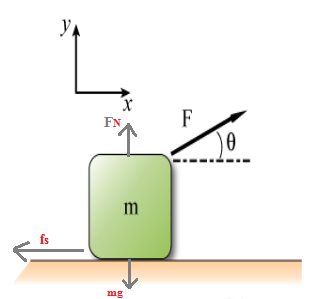(a) The magnitude of acceleration of the block across the floor which is given as :

from a free body diagram, we have

on the y-direction :     Fapp sin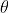+ FN = Fg

Fapp sin 240 + FN = mg

FN = mg - (0.55 mg) sin 240

FN = mg [1 - (0.55) sin 240]                                                          { eq.1 }

on the x-direction :        Fapp cos- Ff = Fx

Fapp cos 240 -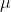k FN = m a                                                          { eq.2 }

(0.55 mg) cos 240 -k mg [1 - (0.55) sin 240] = m a

using,k = 0.505

(because, the block is initially stationary and required a force greater than the maximum force of kinetic friction)

a = (0.55 g) cos 240 - (0.505) g [1 - (0.55) sin 240]

a = (0.55) (9.8 m/s2) (0.9135) - (0.505) (9.8 m/s2) [1 - (0.55) (0.4067)]

a = (4.92 m/s2) - (3.84 m/s2)

a = 1.08 m/s2

(b) The magnitude of acceleration of the block across the floor which is given as :

Fapp cos 240 -s FN = m a

(0.55 mg) cos 240 -s mg [1 - (0.55) sin 240] = m a

using,s = 0.395

(because, the block is initially stationary and required a force greater than the maximum force of static friction)

a = (0.55 g) cos 240 - (0.395) g [1 - (0.55) sin 240]

a = (0.55) (9.8 m/s2) (0.9135) - (0.395) (9.8 m/s2) [1 - (0.55) (0.4067)]

a = (4.92 m/s2) - (3 m/s2)

a = 1.92 m/s2

#### Earn Coins

Coins can be redeemed for fabulous gifts.

Similar Homework Help Questions
• ### The figure below shows an initially stationary block of mass m on a floor. A force...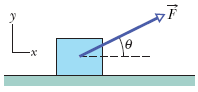The figure below shows an initially stationary block of mass m on a floor. A force of magnitude F = 0.550mg is then applied at upward angle θ = 23°. (a) What is the magnitude of the acceleration of the block across the floor if the friction coefficients are μs = 0.590 and μk = 0.495? m/s2 (b) What is the magnitude of the acceleration of the block across the floor if the friction coefficients are μs = 0.395 and...

• ### The figure shows an initially stationary block of mass m on a floor. A force of...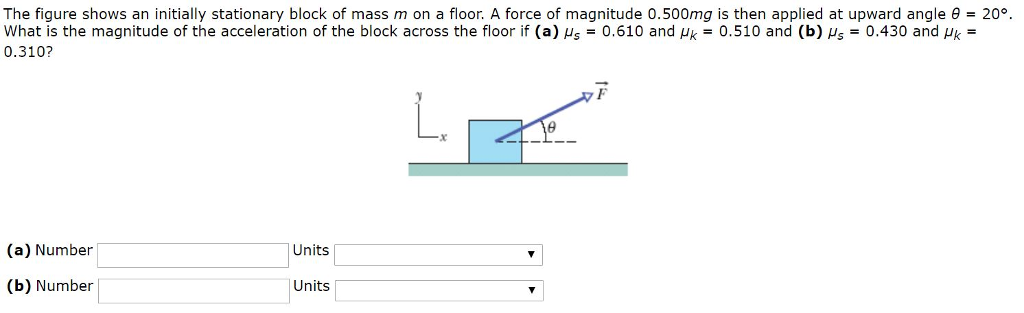The figure shows an initially stationary block of mass m on a floor. A force of magnitude 0.500mg is then applied at upward angle θ = 20°. What is the magnitude of the acceleration of the block across the floor if (a) μs = 0.610 and μk = 0.510 and (b) μs = 0.430 and μk = 0.310? The figure shows an initially stationary block of mass m on a floor. A force of magnitude 0.500mg is then applied at...

• ### The figure below shows an initially stationary block of mass m on a floor. A force...

The figure below shows an initially stationary block of mass m on a floor. A force of magnitude F = 0.540·mg is then applied at upward angle θ = 20°. 6-24.gif (a) What is the magnitude of the acceleration of the block across the floor if (a) μs = 0.600 and μk = 0.500? (b) What is the magnitude of the acceleration of the block across the floor if μs = 0.400 and μk = 0.300? would like steps and...

• ### The figure shows an initially stationary block of mass m on a floor. A force of...

The figure shows an initially stationary block of mass m on a floor. A force of magnitude 0.500mg is then applied at upward angle θ = 20°. What is the magnitude of the acceleration of the block across the floor if (a) μs = 0.630 and μk = 0.530 and (b) μs = 0.420 and μk = 0.340?

• ### An initially stationary block of mass m is on the floor. A force ofmagnitude 0.500mg is...

An initially stationary block of mass m is on the floor. A force ofmagnitude 0.500mg is then applied at upward angle θ = 20°.What is the magnitude of the acceleration of the block across thefloor if (a)μs = 0.640 and μk = 0.510 and (b)μs =0.410 and μk = 0.340? Already found Part A. Don't know what equation to use for part B.If µ is involved in the equation, do you use µs orµk?

• ### The figure below shows an initially stationary block of mass m on a floor. A force...

The figure below shows an initially stationary block of mass m on a floor. A force of magnitude F = 0.600·mg is then applied at upward angle ? = 18°. (a) What is the magnitude of the acceleration of the block across the floor if (a) µs = 0.600 and µk = 0.500? _ m/s2 (b) What is the magnitude of the acceleration of the block across the floor if µs = 0.400 and µk = 0.300? _ m/s2

• ### The figure below shows an initially stationary block of mass m on a floor. A force...

The figure below shows an initially stationary block of mass m on a floor. A force of magnitude F = 0.520·mg is then applied at upward angle ? = 16°. (a) What is the magnitude of the acceleration of the block across the floor if (a) µs = 0.600 and µk = 0.500? m/s2 (b) What is the magnitude of the acceleration of the block across the floor if µs = 0.400 and µk = 0.300? m/s2

• ### Figure 6-20 shows an initially stationary block of mass m on a floor. A force of...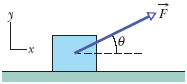Figure 6-20 shows an initially stationary block of mass m on a floor. A force of magnitude 0.500mg is then applied at upward angle ? = 20°. What is the magnitude of the acceleration of the block across the floor if (a)µs = 0.620 and µk = 0.540 and (b)µs = 0.420 and µk = 0.330?

• ### magnitude 7. An initially stationary block of mass m is on a floor. A force of...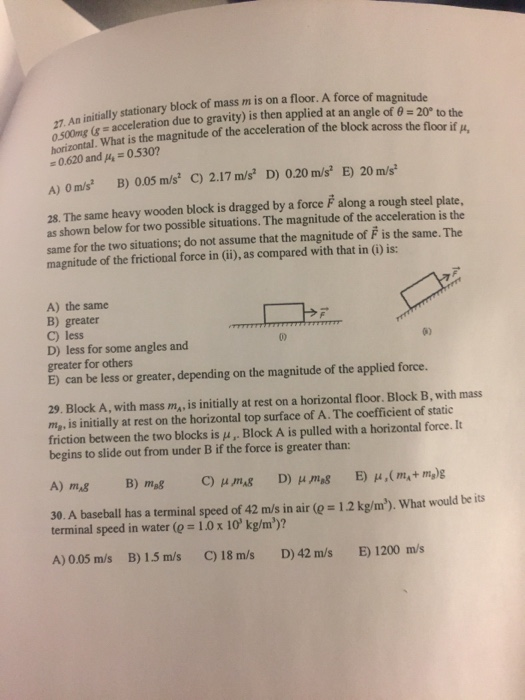magnitude 7. An initially stationary block of mass m is on a floor. A force of acceleration due to gravity) is then applied at an angle of θ-20° to the oseontal, What is the magnitude of the acceleration of the block across the floor if p, 0.620 and p 0530 A) Om/s B) 005 m/s C) 2.17 m/s D) 0.20 m/s' E) 20 m/s 28. The same heavy wooden block is dragged by a force F along a rough steel...

• ### The figure shows an initially stationary block of mass m on a noor. A force of...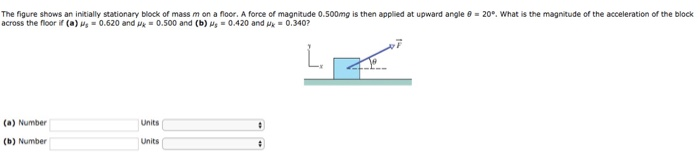The figure shows an initially stationary block of mass m on a noor. A force of magnitude 0.500mg is then applied at upward angle θ·20®. what is the magnitude of the acceleration of the block across the noor if (a) us-0.620 and".-0.500 and (b) 0.420 and M-0340? (a) Number Units (b) Number Units